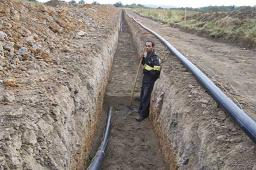# Excavation

Mr. Billy calculated that excavation for a water connection digs for 12 days. His friend would take 10 days. Billy worked 3 days alone. Then his friend came to help and started on the other end.

On what day since the beginning of the excavation they met?

n =  8

### Step-by-step explanation:

3 / 12 +(n-3)/12 + (n-3)/10 = 1

11n = 78

n = 78/11 ≈ 7.090909

Our simple equation calculator calculates it.Did you find an error or inaccuracy? Feel free to write us. Thank you!Tips to related online calculators
Check out our ratio calculator.
Do you have a linear equation or system of equations and looking for its solution? Or do you have a quadratic equation?
Do you want to round the number?
Do you want to convert time units like minutes to seconds?